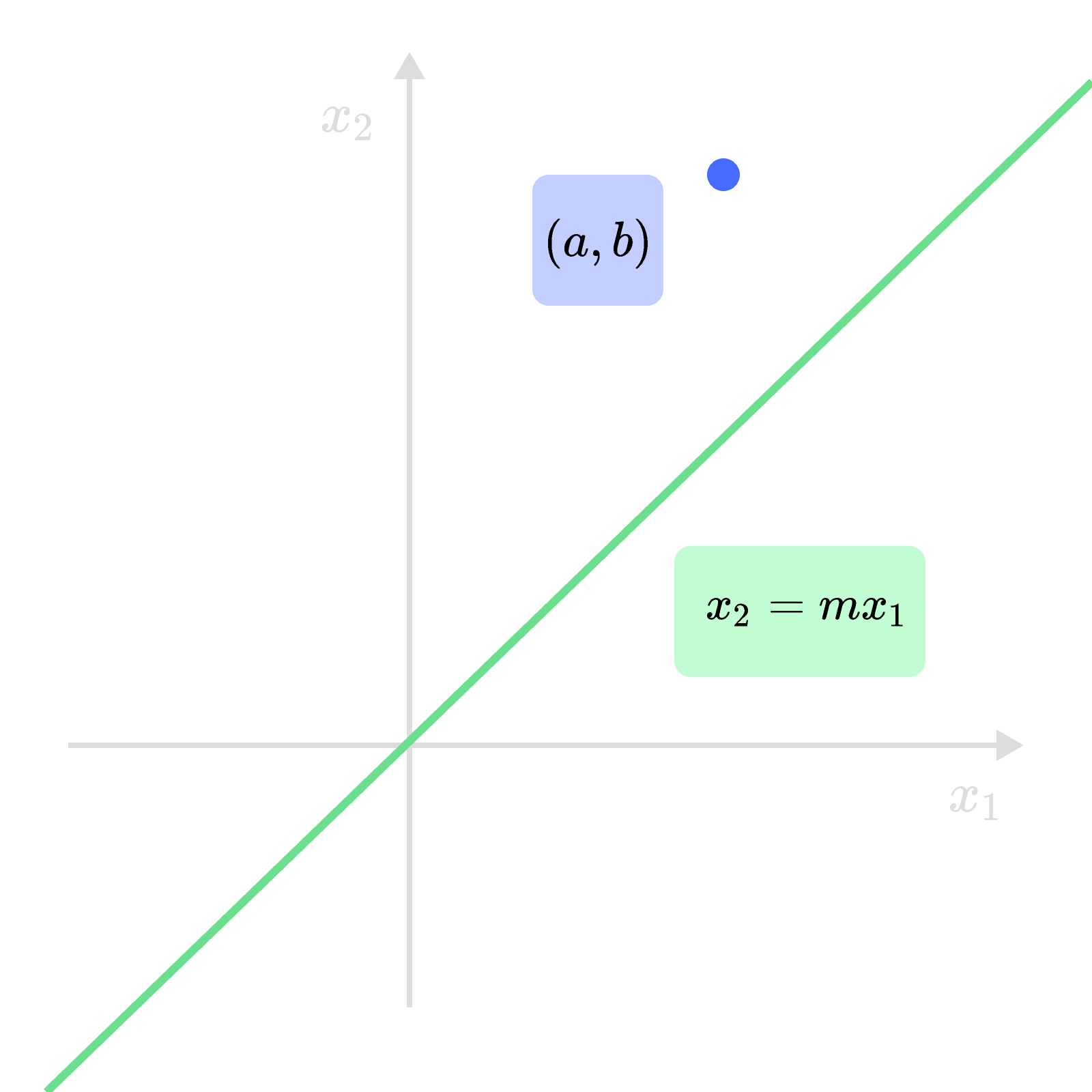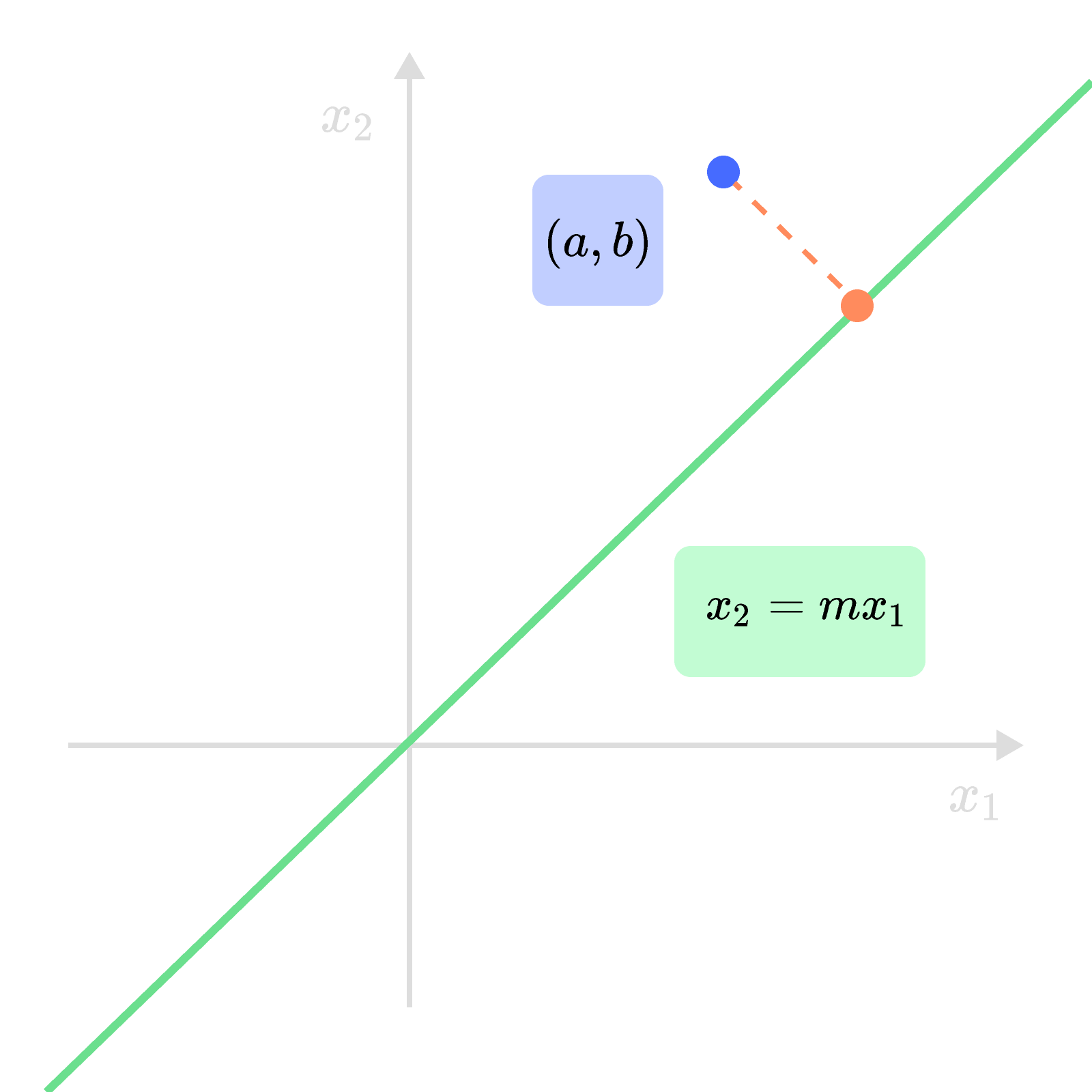### Linear Algebra with Applications

Waves have a lot of nice properties that make them easy to analyze.

So when a scientist or engineer needs to analyze a signal that's not exactly a wave, they often approximate it as a wave.

It turns out that vector spaces show us the "best" possible way to do this!

In this quiz, we'll sketch the basic ideas and fill the details in later.

Note on Notation:

$(f+g)(t)$ means "evaluate $f +g$ at $t$," not "multiply $f + g$ by $t.$"

# Why Vector Spaces?

Any signal of interest to a scientist is a real-valued function $f$ of time $t.$ Let's call the set of all such functions $F.$

Waves live in $F,$ too, but they're special since they repeat. Put all the waves, i.e. functions obeying $w(t+1) = w(t),$ in a subset called $W.$

We can add functions and scalar-multiply with the following rules: $(f+g)(t) = f(t) + g(t) \hspace{0.5cm} \text{and} \hspace{0.5cm} (c f)(t) = c f(t).$

Which of the following statements is true?

Note: For reference, you can find the definition of a vector space here.

# Why Vector Spaces?

Both $F$ and $W$ are vector spaces, so we can rephrase our original problem of approximating a signal by a wave this way:

What vector in the subspace $W$ is as close to $|f\rangle \in F$ as possible?

We call $W$ a subspace because it is a space that's also a subset.

There's a way of solving this problem based solely on vector ideas. That means we can develop a strategy for solving it in, say, the plane (which is easier to visualize) and carry what we learn there over to the space $F!$

# Why Vector Spaces?

In the picture below, the point plays the role of the signal, the line plays the role of $W,$ and the Cartesian plane corresponds to $F.$

Before we can take the analogy too seriously, we have to prove that the Cartesian plane is, in fact, a vector space.

The plane is made up of all real number pairs $(x_{1},x_{2}),$ so we can define addition and scalar multiplication naturally as \begin{aligned} (x_{1} , x_{2}) + (y_{1} , y_{2}) & = (x_{1} +y_{1}, x_{2} + y_{2}) \\ c (x_{1},x_{2}) & = (c x_{1} , c x_{2} ). \end{aligned} With these definitions, is the Cartesian plane a vector space?# Why Vector Spaces?

With $(x_{1} , x_{2} ) + (y_{1},y_{2})= (x_{1}+y_{1}, x_{2} + y_{2})$ and $c(x_{1},x_{2}) = (c x_{1}, c x_{2} ),$ the Cartesian plane is a vector space.

As a set, the line in the picture below consists of all pairs $(x_{1}, m x_{1} )$ where $m$ is the slope.

It inherits addition and scalar multiplication from the plane, but to be a vector subspace, it has to be closed under these operations. This means

• the sum of any two points on the line is another point on the line;
• scaling a point on the line produces another point on the line.

Select the correct statement from the list of options.# Why Vector Spaces?

So far, our analogy works quite well: both the Cartesian plane $($playing the role of $F)$ and the line $($playing the role of $W)$ are vector spaces.

Now, we need to decide how to tell if the point $(a,b)$ (representing the signal) is "close" to a point $(x_{1} , m x_{1} )$ on the line.

The natural way to do this is to use the distance between $(a,b)$ and $(x_{1} , m x_{1} ),$ which is the length of the line segment connecting them.

The interactive below lets you pick a point on the line with slope $m =0.5.$ It then computes the distance between it and $(a,b) = (2,3).$

(We choose these numbers just to be concrete!)

Is there a smallest possible distance?

# Why Vector Spaces?

With a bit more work, we can actually show that the line segment minimizing the distance is perpendicular to the original line.So here's the strategy we should import to the signal problem:

• Define distances between vectors in $F$ and $W. \\\\ \text{} \\\\$
• Minimize the distance from $W$ to the signal $|f \rangle \in F.$

It'll take some work, but in later chapters we'll show there's a purely vector-based way to do these things!

# Why Vector Spaces?

We can't fill in the details here, but we can certainly peek ahead and see the result of applying this strategy to a slightly simpler problem.

Suppose instead that $F$'s functions are defined on the smaller interval $[0,1],$ and $W$ is built up from the waves $\big | \sin\left( 2 \pi t \right) \big\rangle , \big | \sin\left( 4 \pi t \right) \big\rangle , \big | \sin\left(6 \pi t \right) \big\rangle , \ldots, \big | \sin\left( 2 \pi n t \right) \big\rangle,$where $n$ is a positive integer.

The interactive below shows the result of approximating a step function by a combination of these sine waves:

The more waves we use, the better, but even with just a few waves the signal approximation is pretty good.

The scheme we outlined in this quiz applies to many real-world problems from physics and statistics, as we'll see.

This is just one example of how useful the vector space concept can be.

×